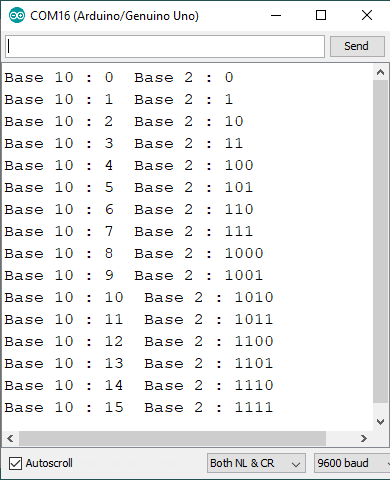# Byte variable in Arduino

In this topic we will see how to use byte variable in Arduino. Generally we use base 10 in our daily life for computing/counting, but microcontrollers and/or processors do computing using base 2 or Binary number system. For an example 255 (27 + 26 + 25 + 24 + 23 + 22 + 21 + 20 = 128 + 64 + 32 + 16 + 8 + 4 + 2 + 1) of decimal will be written as (11111111)2

Way to store a binary number is by using Byte variable in same manner we use data types such as int, float, char

Syntax:                 byte var_name = B11111111;

This B prefix tells the arduino to read the number in binary form not in decimal number system because

(11111111)2 ≠ (11111111)10

### PROGRAMMING CODE – BYTE VARIABLE

```byte a;
void setup() {
Serial.begin(9600);
}
void loop() {
for (int i = 0 ; i <= 15 ; i++) {
a = i;
Serial.print("Base 10 : ");
Serial.print(a, DEC);    // DEC is use to print value of a in DECIMAL Number
Serial.print("  Base 2 : ");
Serial.println(a, BIN);    // BIN is use to print value of a in BINARY Number
delay(1000);
}
while (1);
}```

#### OUTPUTSHIFT REGISTER IC 74HC595

5 1 vote
Article Rating
Subscribe
Notify of